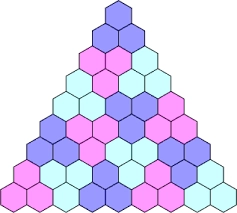# Sequence 80450

How many terms does the sequence have if a1=4, Sn=589, d=3, n=?

n =  19

### Step-by-step explanation:Did you find an error or inaccuracy? Feel free to write us. Thank you!

Tips for related online calculators
Are you looking for help with calculating roots of a quadratic equation?
Need help calculating sum, simplifying, or multiplying fractions? Try our fraction calculator.
Do you have a linear equation or system of equations and looking for its solution? Or do you have a quadratic equation?

#### Grade of the word problem:

We encourage you to watch this tutorial video on this math problem: